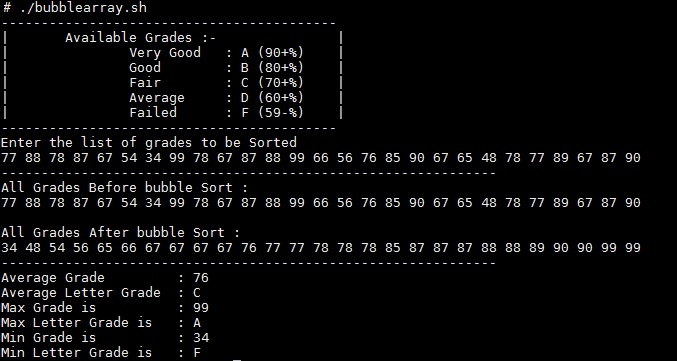# Grade calculation in shell script | Shell Scripting

If you are trying to learn bash scripting and want to learn arithmetic operations and arrays in bash then this example will be helpful to you.

Here we are using bash scripting to calculate average, minimum and maximum grade and its subsequent letter grade using bubble sorting. Bubble sort, sometimes referred to as sinking sort, is a simple sorting algorithm that works by repeatedly stepping through the list to be sorted, comparing each pair of adjacent items and swapping them if they are in the wrong order. The pass through the list is repeated until no swaps are needed, which indicates that the list is sorted. If you are sorting the data in Ascending order, at the end of the first pass, the  “heaviest” element has move to bottom. In the second pass, the comparisons are made till the last but one position and now second largest element is placed at the last but one position. And so forth.

Below is the script written in bash:
Script Name: bubblearray.sh

```#!/bin/bash
#####################################################################
# Define Functions Here #
#####################################################################

printnumbers()
{
echo \${ARRAY[*]}

#You can also use bellow code
#for ((i=0;i<count;i++))
#do
#echo -n " \${ARRAY[i]} "
#done
}

exchange()
{
temp=\${ARRAY[\$1]}

ARRAY[\$1]=\${ARRAY[\$2]}

ARRAY[\$2]=\$temp

}

sortnumbers()
{
for (( last=count-1;last>0;last--))
do
for((i=0;i<last;i++))
do
j=\$((i+1))
if [ \${ARRAY[i]} -gt \${ARRAY[j]} ]
then

exchange \$i \$j

fi
done
done
}

#Checking the overall grade according to average marks scored.

gradecalc(){

ifgrade=\$1

if [[ \$ifgrade -ge 90 && \$ifgrade -le 100 ]]; then
echo "A"

elif [[ \$ifgrade -ge 80 && \$ifgrade -le 89 ]]; then

echo "B"

elif [[ \$ifgrade -ge 70 && \$ifgrade -le 79 ]]; then
echo "C"
elif [[ \$ifgrade -ge 60 && \$ifgrade -le 69 ]]; then
echo "D"

elif [[ \$ifgrade -ge 0 && \$ifgrade -le 59 ]]; then
echo "F"
else
echo "Incorrect Value Entered"

fi
}
#####################################################################
# Variable Initialization #
#####################################################################

echo "------------------------------------------";
echo "| Available Grades :- |";
echo "| Very Good : A (90+%) |";
echo "| Good : B (80+%) |";
echo "| Fair : C (70+%) |";
echo "| Average : D (60+%) |";
echo "| Failed : F (59-%) |";
echo "------------------------------------------";

echo "Enter the list of grades to be Sorted"

read -a ARRAY

count=\${#ARRAY[@]}

#####################################################################
# Main Script Starts Here #
#####################################################################

echo "--------------------------------------------------------------"

echo "All Grades Before bubble Sort :"

printnumbers

echo

sortnumbers

echo "All Grades After bubble Sort : "

printnumbers

echo "--------------------------------------------------------------"

sum=\$(
awk 'BEGIN {t=0; for (i in ARGV) t+=ARGV[i]; print t}' "\${ARRAY[@]}"
)

#echo -ne "Sum of Array Numbers : \$sum \n"

# Finding Array length to calculate the average grade

tLen=\${#ARRAY[@]}

#calculating the average

decimalavg=\$(echo \$sum / \$tLen | bc -l)

#echo -ne "Decimal Average: \$decimalavg \n";

#Rounding off the average.

avg=`echo "(\$decimalavg+0.5)/1" | bc`

echo -en "Average Grade : \$avg \n";

echo -en "Average Letter Grade : \$(gradecalc \${avg}) \n";

# finding min/max
max=\${ARRAY}
min=\${ARRAY}

# Loop through all elements in the array
for i in "\${ARRAY[@]}"
do
# Update max if applicable
if [[ "\$i" -gt "\$max" ]]; then
max="\$i"
fi

# Update min if applicable
if [[ "\$i" -lt "\$min" ]]; then
min="\$i"
fi
done

# Output results:
echo "Max Grade is : \$max";
echo "Max Letter Grade is : \$(gradecalc \${max}) ";
echo "Min Grade is : \$min"
echo "Min Letter Grade is : \$(gradecalc \${min}) ";
```

Sample Outputs:In case of any ©Copyright or missing credits issue please check CopyRights page for faster resolutions.

This site uses Akismet to reduce spam. Learn how your comment data is processed.